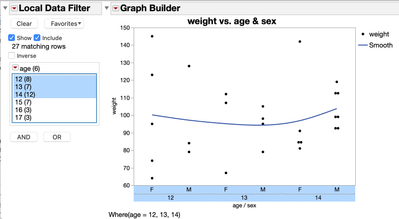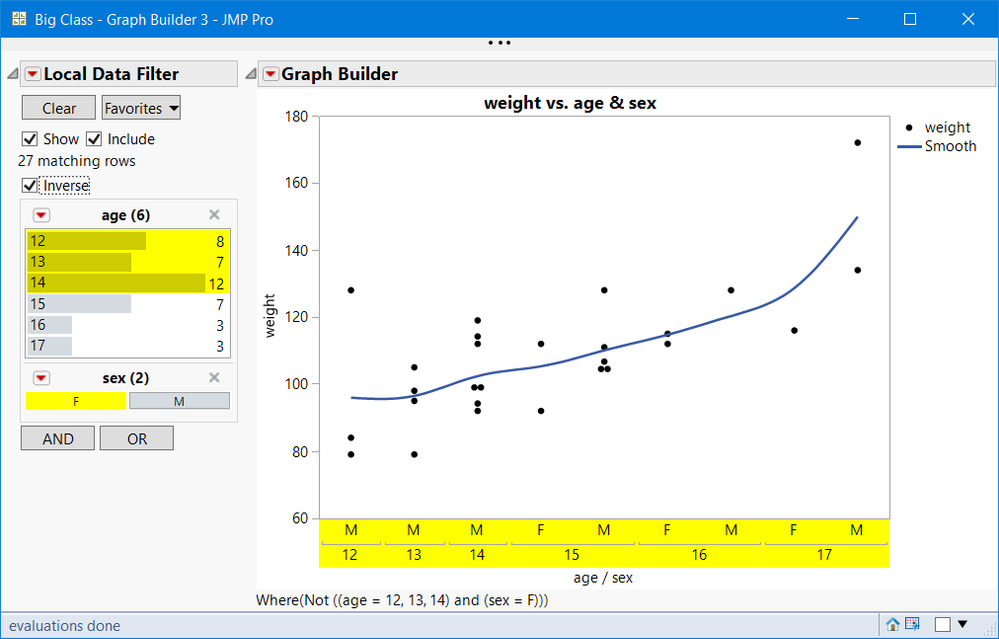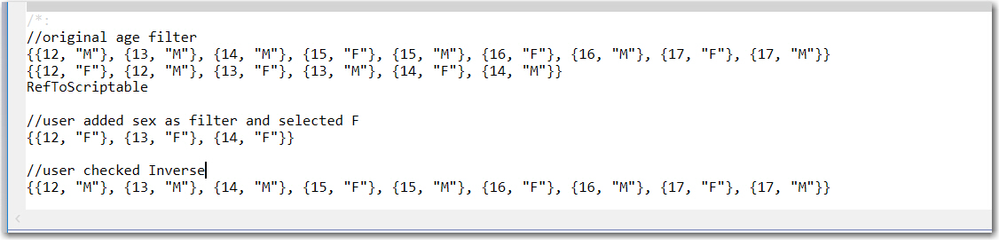Our World Statistics Day conversations have been a great reminder of how much statistics can inform our lives. Do you have an example of how statistics has made a difference in your life? Share your story with the Community!
Choose Language Hide Translation Bar
Highlighted

## Is there a method to get axis values from graph builder?

Hi.  I'm interested in knowing there is a scripting method to read the displayed values for an axis in graphbuilder and return them as a list.  IOW, is there a function or a message that can "get" the axis values from a graphbuilder reference or an axis box?  Something like: xval_lis = gb << Get X Axis Values.

For instance, suppose I have the following graph:``````dt = Open("\$SAMPLE_DATA/Big Class.jmp");
gb = Graph Builder(
Size(513, 406),
Show Control Panel(0),
Back Color("White"),
Variables(X(:age), X(:sex, Position(1)), Y(:weight)),
Elements(Points(X(1), Y, Legend(1)), Smoother(X(1), Y, Legend(2))),
Local Data Filter(
columns(:age),
Where(:age == {12, 13, 14}),
Display(:age, Size(170, 96), List Display)
)
),
SendToReport(Dispatch({}, "", AxisBox, {Select}))
)``````

Here, since the user has excluded certain ages using the local data filter, not all of the ages in the table are shown in x-axis.  In this case I would like a way to read off which ages are actually displayed in the graph.  This question applies only when a nominal or ordinal-type variable is used for the axis (no continuous variables).

I've considered the following scripting method:

1. Read the local data filter

2. Create a subset table of just the unfiltered rows

3. Use the Summarize function on that table to get my list of displayed axis values

``````ldf_str = ldf << Get Where Clause;  //ldf = reference to local data filter in gb window
clause = Parse(Regex(ldf_str, "Select Where\((.+)\)\$", "\1"));
r = dt << Get Rows Where(Eval(clause));   //dt = ref to main datable
dtsub = dt << Subset(//Invisible,
Selected Rows(0),
Rows(r),
Selected columns only(0)
);
Summarize(dtsub, xlev_lis = By(:age, :sex));``````

This method seems unwieldy though.  Is there a command that can "get" the axis values from a graphbuilder reference or an axis box?

1 ACCEPTED SOLUTION

Accepted Solutions
Highlighted

## Re: Is there a method to get axis values from graph builder?

Not sure of your user scenario, however, if the user is actively filtering, they can also change the GraphBuilder structure, different roles, etc. So a Filter Change Handler would be  more robust. Also note the Where Clause() does not capture the Inverse. The script and the TextBox of the Where captures the Inverse as a Not (!)Below and attached is a script that gets the Xticks and prints them to the Log when the filter changes.  Here is a screen shot of the embedded Log with comments (//) and extra line feeds.``````Names Default to Here(1);

dt = Open("\$SAMPLE_DATA/Big Class.jmp");
gb = Graph Builder(
Size(513, 406),
Show Control Panel(0),
Back Color("White"),
Variables(X(:age), X(:sex, Position(1)), Y(:weight)),
Elements(Points(X(1), Y, Legend(1)), Smoother(X(1), Y, Legend(2))),
Local Data Filter(
columns(:age),
Where(:age == {12, 13, 14}),
Display(:age, Size(170, 96), List Display)
)
),
SendToReport(Dispatch({}, "", AxisBox, {Select}))
);
//iftb = (report(gb)[PictureBox(1)]<<sib)[TextBox(1)]; //get handle to the textbox
ldf = (gb<<parent)<<child;
gbb = report(gb)[GraphBuilderBox(1)] ;

f = function({a}, {zz,aa1,varlist={}, _xvar_={}, i, _xticks={}},
varlist = gbb << get variables;
for(i=1, i<=nitems(varlist), i++,
if(nameExpr(varlist[i]) == nameExpr(Role( "X" )), insertinto(_xvar_, varlist[i] <<get name) );
);
zz= ldf << get filtered rows;
if(isMissing(zz), zz= Index(1, nrow(dt)) );
//check for excluded rows
_excl = dt << get excluded rows;
aa1 = AssociativeArray(zz);
aa1 = Remove(aa1, Aslist(_excl));
zz  = aa1<<get keys;
//zz are the rows used in creating the graph

//now use the Associative Array to get unique values
_xticks = Associative Array( dt[zz,_xvar_], AsList(J(nitems(zz),1,1)) ) << get keys;
print(_xticks);  //see the log
);
f(1);
rs = ldf << Make Filter Change Handler( f );

``````

Notes:

• Line 20 is commented; it shows how to get a handle to the Where TextBox.
• Key features of the function f used for the Filter Change Handler are the capabilties of the JMP implementation of Associative Array: multiple methods to define it;  to get unique values in a list; almost any JMP object can be used as a key.
• Other key features are:  dt[ zz, _xvar_] is a list of row values, and ldf << get filtered rows.

2 REPLIES 2
Highlighted

## Re: Is there a method to get axis values from graph builder?

Not sure of your user scenario, however, if the user is actively filtering, they can also change the GraphBuilder structure, different roles, etc. So a Filter Change Handler would be  more robust. Also note the Where Clause() does not capture the Inverse. The script and the TextBox of the Where captures the Inverse as a Not (!)Below and attached is a script that gets the Xticks and prints them to the Log when the filter changes.  Here is a screen shot of the embedded Log with comments (//) and extra line feeds.``````Names Default to Here(1);

dt = Open("\$SAMPLE_DATA/Big Class.jmp");
gb = Graph Builder(
Size(513, 406),
Show Control Panel(0),
Back Color("White"),
Variables(X(:age), X(:sex, Position(1)), Y(:weight)),
Elements(Points(X(1), Y, Legend(1)), Smoother(X(1), Y, Legend(2))),
Local Data Filter(
columns(:age),
Where(:age == {12, 13, 14}),
Display(:age, Size(170, 96), List Display)
)
),
SendToReport(Dispatch({}, "", AxisBox, {Select}))
);
//iftb = (report(gb)[PictureBox(1)]<<sib)[TextBox(1)]; //get handle to the textbox
ldf = (gb<<parent)<<child;
gbb = report(gb)[GraphBuilderBox(1)] ;

f = function({a}, {zz,aa1,varlist={}, _xvar_={}, i, _xticks={}},
varlist = gbb << get variables;
for(i=1, i<=nitems(varlist), i++,
if(nameExpr(varlist[i]) == nameExpr(Role( "X" )), insertinto(_xvar_, varlist[i] <<get name) );
);
zz= ldf << get filtered rows;
if(isMissing(zz), zz= Index(1, nrow(dt)) );
//check for excluded rows
_excl = dt << get excluded rows;
aa1 = AssociativeArray(zz);
aa1 = Remove(aa1, Aslist(_excl));
zz  = aa1<<get keys;
//zz are the rows used in creating the graph

//now use the Associative Array to get unique values
_xticks = Associative Array( dt[zz,_xvar_], AsList(J(nitems(zz),1,1)) ) << get keys;
print(_xticks);  //see the log
);
f(1);
rs = ldf << Make Filter Change Handler( f );

``````

Notes:

• Line 20 is commented; it shows how to get a handle to the Where TextBox.
• Key features of the function f used for the Filter Change Handler are the capabilties of the JMP implementation of Associative Array: multiple methods to define it;  to get unique values in a list; almost any JMP object can be used as a key.
• Other key features are:  dt[ zz, _xvar_] is a list of row values, and ldf << get filtered rows.

Highlighted

## Re: Is there a method to get axis values from graph builder?

Thanks @gzmorgan0. It took a little time for me to grasp, but this is a nice solution. I appreciate the help!
Article Labels